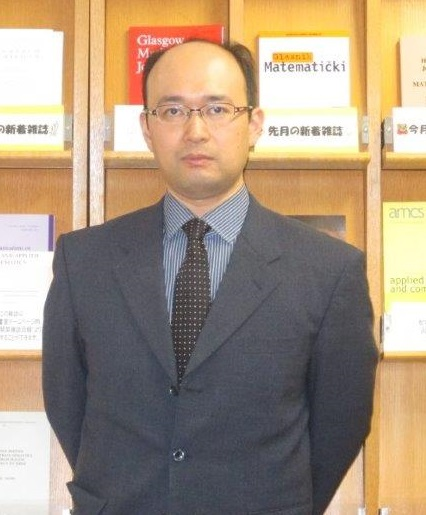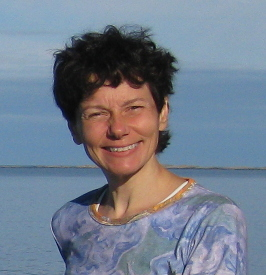# Tutorial Speakers

## Titles and Abstracts

Shaoshi Chen### A Reduction Approach to Creative Telescoping

Abstract:

Creative telescoping is the core in the algorithmic proof theory of combinatorial identities developed by Wilf and Zeilberger in the early 1990s. The process of creative telescoping constructs linear differential or recurrence operators in one variable for multivariate functions. Such operators are called telescopers.

Four classes of algorithms have been developed for creative telescoping according to different algorithmic techniques on which they are based. The fourth and most recent one is the reduction-based telescoping algorithms that are based on Hermite reduction and its variants. Algorithms in this class share the common feature that they separate the computation of telescopers from the costly computation of certificates. This idea was first worked out for bivariate rational functions in 2010. It has since been extended to more general classes of functions, such as hyperexponential functions, hypergeometric terms, algebraic functions and most recently D-finite functions.

In this tutorial, we will overview several reduction algorithms in symbolic integration and summation, explain the idea of creative telescoping via reductions, and present intriguing applications of this new approach.

Satoshi Aoki
Kobe University, Japan.### Algebraic methods in a design of experiments.

Abstract:

Applications of Groebner basis theory to various problems of statistics arises in early 1990s. One of the first works in this field, a computational algebraic statistics, is given by Pistone and Wynn (1996, Biometrika), where the Groebner basis theory is applied to the identifiability problem in the design of experiments. After this work, various algebraic approaches to the problems in the design of experiments are presented by researchers both in the fields of algebra and statistics.

In this tutorial, starting with a fundamental theory of statistics on the design and analysis of experiments, we will overview algebraic methods in this field.

Anna M. Bigatti
Università Degli Studi Di Genova, Italy.### Linear Algebra for Zero-Dimensional Ideals

Abstract:

Given a zero-dimensional ideal I in a polynomial ring, many algorithms start by finding univariate polynomials in I, or by computing a lex-Groebner basis of I. These are related to considering the minimal polynomial of an element in P/I, which may be computed using Linear Algebra.

In this tutorial we'll see algorithms for computing minimal polynomials, especially for the case of rational coefficients, and then some applications, namely algorithms for computing radicals and primary decompositions of zero-dimensional ideals, and also for testing radicality and maximality.

All this will be done with a special eye on the practical implementation, and with demostrations in CoCoA.